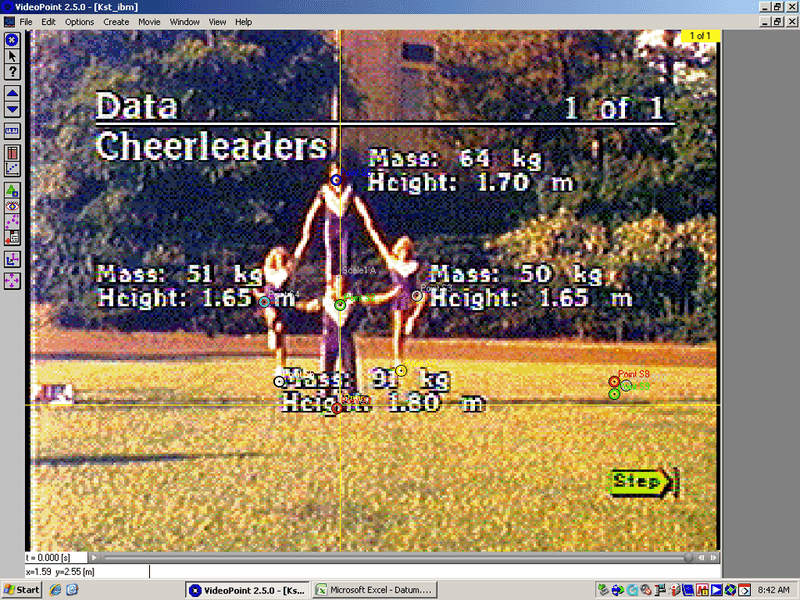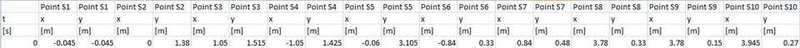# Simple Force problem(Picture and Graphical Data included.)

Hey, I've just started the curriculum at my school and can not seem to figure this problem out.

## Homework Statement

Basically, I have the image below of a few cheerleaders doing a pose. They are in complete equilibrium. I have to find the force put on where the top cheerleader's hands are connected to the top cheerleader. I have to find the force put on by the bottom cheerleader to the guys on the sides. I have to find the force that is being put on the bottom guy's shoulder. Also, what is the force that is being put onto the bottom guy's feet. My points are also attached.## Homework Equations

Force = Mass x Acceleration of Gravity.
Pythagorean Theorem.

## The Attempt at a Solution

I think that the force that is being put on the top cheerleader from the two guys to the sides is similar to the simple conversion of each of the masses of the cheerleaders time 9.81(the acceleration of gravity.) Now, would I use the value that I get that is the perpendicular to the floor, or would I use the force that has a vertical length. This would just be for the hands. Now, to get the force that the person is putting onto the bottom person from the sides, I though that I would take the mass of the cheerleaders on the sides and figure the horizontal length in newtons. The

This problem has stumped me. Thanks in advance, if someone may help me out.

Last edited: